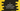# C program to find the sum of A.P. series# Introduction :

A.P. or arithmetic progression series is a sequence of numbers in which each number is the sum of the preceding two numbers. For example, 2, 3, 5, 8, 13… is an A.P. or arithmetic progression series.

In this tutorial, we will learn how to find the sum of A.P. series in C programming language.

## Formula to find the sum :

We have two main formula for an A.P. series :

``nth element = a + (n-1)*d``

Here, a is the start element of the series. n is the element number. d is the common difference.

For example, for common difference 3, first element : 1 second element : 1 + (2-1)3 = 1 + 3 = 4 third element : 1 + (3-1)3 = 1 + 6 = 7 fourth element : 1 + (4-1)3 = 1 + 9 = 10 fifth element : 1 + (5-1)3 = 1 + 12 = 13

1, 4, 7, 10, 13… is an A.P. series.

The sum of the first n items is :

``n/2(2a + (n-1) d)``

for A.P. 1, 4, 7, 10, 13…, the sum of the first 5 numbers is :

``5/2(2*1 + (5-1)*3) = 5/2 * (2 + 12) = 5/2 * 14 = 35 ``

## C program :

The C program will take the first element, total elements and common difference from the user.

``````#include <stdio.h>

int main()
{
int n, a, diff;
int i;
int sum;

printf("Enter the first element of the A.P. series : \n");
scanf("%d", &a);

printf("Enter the total element of the A.P. series : \n");
scanf("%d", &n);

printf("Enter the common difference of the A.P. series : \n");
scanf("%d", &diff);

printf("The A.P. series is : \n");
for (i = a; i <= (a + (n - 1) * diff); i += diff)
{
printf("%d ", i);
}

printf("\nSum : ");
sum = (n *(2 * a + ((n - 1) * diff)))/2;
printf("%d ",sum );

return 0;
}``````

### Explanation :

In this example, we have used the same formula explained above. We are also printing the elements in the A.P. with the sum. For printing the elements, we are using one for loop. The program takes the first element, the total number of elements and common difference from the user and prints the values.

### Sample Output :

``````Enter the first element of the A.P. series :
1
Enter the total element of the A.P. series :
5
Enter the common difference of the A.P. series :
3
The A.P. series is :
1 4 7 10 13
Sum : 35

Enter the first element of the A.P. series :
3
Enter the total element of the A.P. series :
4
Enter the common difference of the A.P. series :
6
The A.P. series is :
3 9 15 21
Sum : 48``````

### Conclusion :

As you have seen in this tutorial, finding the sum of A.P. is really easy if we know the formula for that. Try to run the example with different values and drop one comment below if you have any queries.